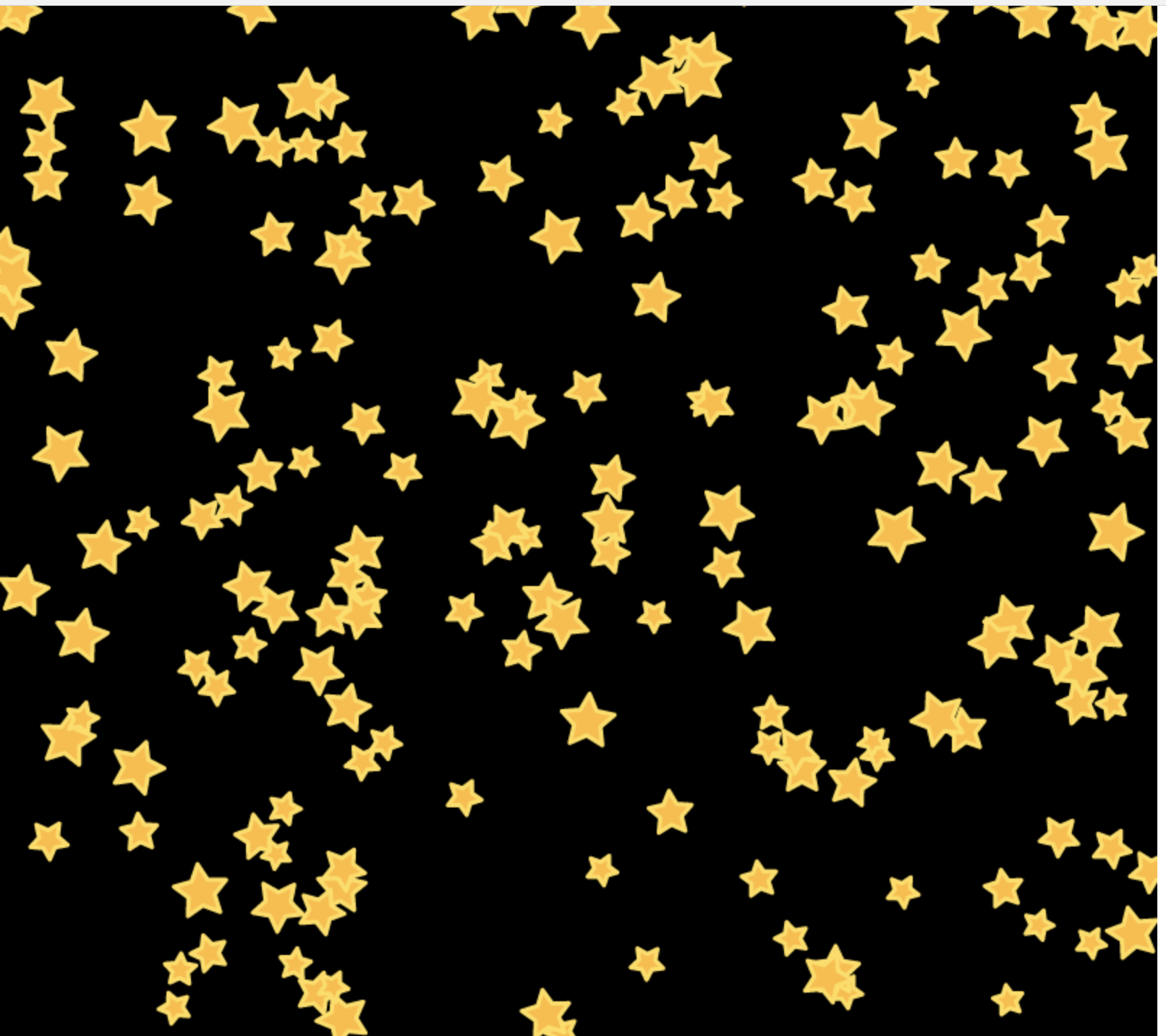# 三角函数在前端动画中的应用

2018/08/26 · JavaScript · 动画## 三角函数JavaScript

sinθ = a / h cosθ = b / h tanθ = a / b

 1 2 3 sinθ = a / h cosθ = b / h tanθ = a / b

JavaScript

r = Math.sqrt(x^2 + y^2) θ = Math.atan2(y, x)

 1 2 r = Math.sqrt(x^2 + y^2) θ = Math.atan2(y, x)A 控制振幅，A 值越大，波峰和波谷越大，A 值越小，波峰和波谷越小；
B 值会影响周期，B 值越大，那么周期越短，B 值越小，周期越长。
C 值会影响图像左右移动，C 值为正数，图像右移，C 值为负数，图像左移。
D 值控制上下移动。

## 一、画一片星空window.onload=function(){ var canvas=document.getElementById("canvas"); canvas.width=800; canvas.height=800; var context=canvas.getContext("2d"); context.fillStyle="black"; context.fillRect(0,0,canvas.width,canvas.height); for(var i=0;i<200;i++){ var r=Math.random()*10+10; var x=Math.random()*canvas.width; var y=Math.random()*canvas.height; var a=Math.random()*360; drawStar(context,x,y,r,r/2.0,a); } } //rot顺时针旋转的角度 function drawStar(ctx,x,y,r,R,rot){ ctx.beginPath(); //角度转弧度：除以180*PI for(var i=0;i<5;i++){ ctx.lineTo(Math.cos((18+i*72-rot)/180*Math.PI)*R+x, -Math.sin((18+i*72-rot)/180*Math.PI)*R+y); ctx.lineTo(Math.cos((54+i*72-rot)/180*Math.PI)*r+x, -Math.sin((54+i*72-rot)/180*Math.PI)*r+y); } ctx.closePath(); ctx.fillStyle="#fb3"; ctx.strokeStyle="#fd5"; ctx.lineWidth=3; ctx.lineJoin="round"; ctx.fill(); ctx.stroke(); } View Code

产生一个扁平化设计中200个星星的效果。## 二、图像变换和状态保存

### 图像应用

JavaScript

for (let x = 0; x < width; x++) { const y = Math.sin(x * a) * amplitude }

 1 2 3 for (let x = 0; x < width; x++) {   const y = Math.sin(x * a) * amplitude }JavaScript

for (let x = 0; x < width; x++) { const radians = x / width * Math.PI * 2 const scale = (Math.sin(radians - Math.PI * 0.5) + 1) * 0.5 const y = Math.sin(x * 0.02 + xSpeed) * amplitude * scale }

 1 2 3 4 5 for (let x = 0; x < width; x++) {   const radians = x / width * Math.PI * 2   const scale = (Math.sin(radians - Math.PI * 0.5) + 1) * 0.5   const y = Math.sin(x * 0.02 + xSpeed) * amplitude * scale }